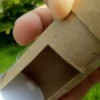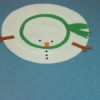# Finding the Center of a Circle

When you need to find the middle of a round project, it helps to know a little geometry. This page is about finding the center of a circle.

### Tip: Finding the Center of a Circle

September 8, 2012

I recently posted the cat tree project. I selected a round base to use for it, but that made it a little harder to find the center than if it had been a square. I thought I would share a quick way to find the center of a circle.

Total Time: 5 minutes

Supplies:

• 1 pre-cut plywood circle
• 1 metal carpenters square

Steps:

1. First you need to select a carpenter's square that is larger than your circle. Place the square so that the point is at the edge of the circle. Take a ruler, lay it across the square so that it lines up with the points where the square and circle edges aline. Then draw a line with your pencil.

3. Next flip your square over so that the point is now touching the opposite edge of the circle. Repeat step one. You will now have two pencil lines that intersect at the center of your circle.

### Video: How to Find the Center of a Circle

September 11, 2012

This video show you the steps to finding the center of a circle. View the full project here: Finding the Center of a Circle

Categories
PagesMore
Categories